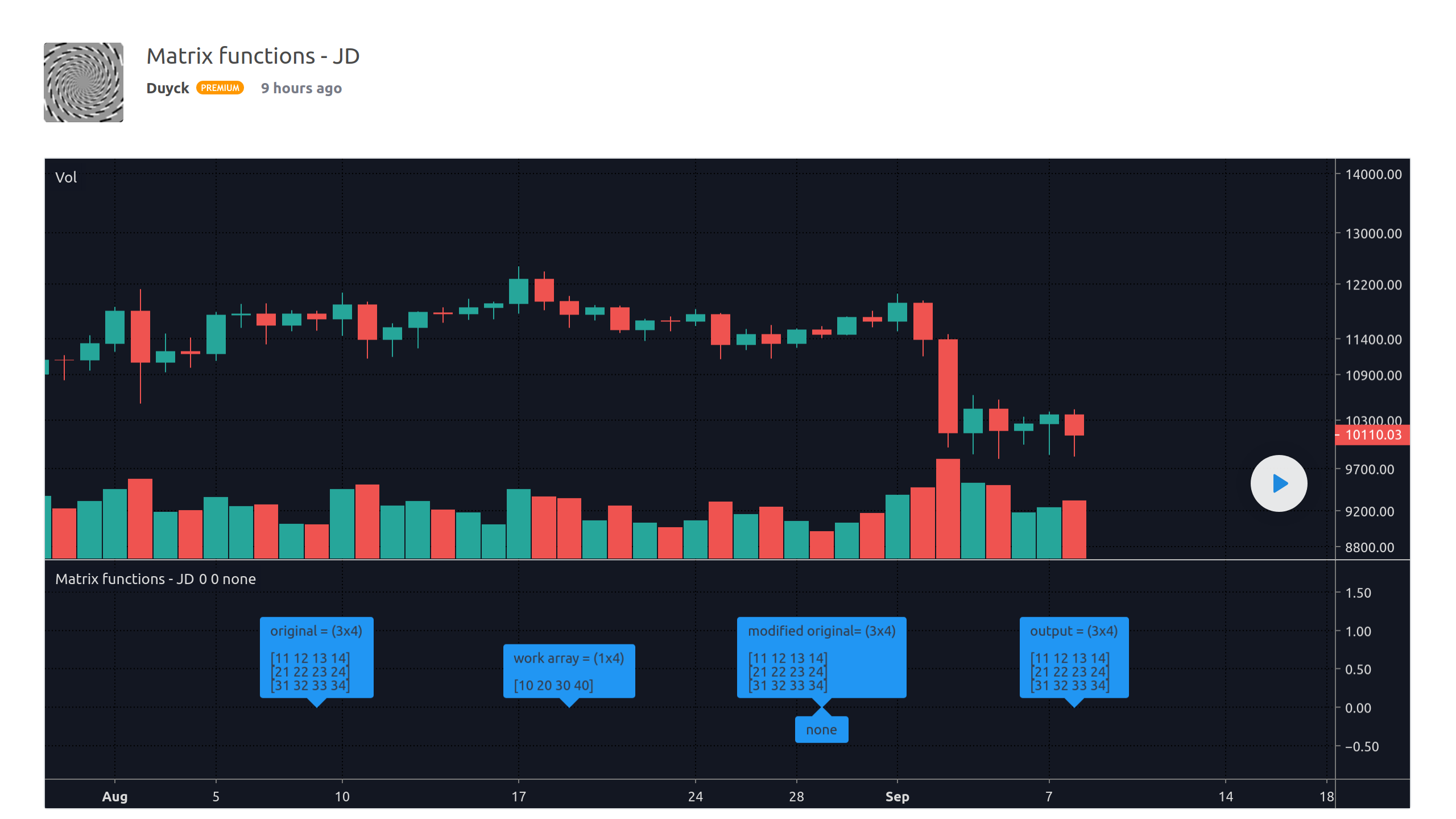September 10, 2020
Pine现在可以使用阵列

Pine脚本现在可以使用强大的新阵列功能来创建自订数据集。阵列允许编程人员在包含以下类型之一的元件的一维资料结构中进行填充、管理、和计算，从而显著扩展了Pine的建模功能：float, int, bool, or color

`levels = array.new_float(size = 3, initial_value = na)`

```//@version=4
study("Price Avg Of Increasing Volume Bars", "", true)
i_levels = input(20,   "Levels")
i_src    = input(hlc3, "Source")

// Initialize array of user-selected size with `na` values.
var levels = array.new_float(i_levels)

if rising(volume, 1)
// Remove the oldest level from the beginning of the array.
array.shift(levels)

// Add the new level to the end of the array.
array.push(levels, i_src)

// Calculate the avg of levels in the array, excluding any `na` values.
level = array.avg(levels)
plot(level, "Level", close > level ? color.lime : color.fuchsia)

```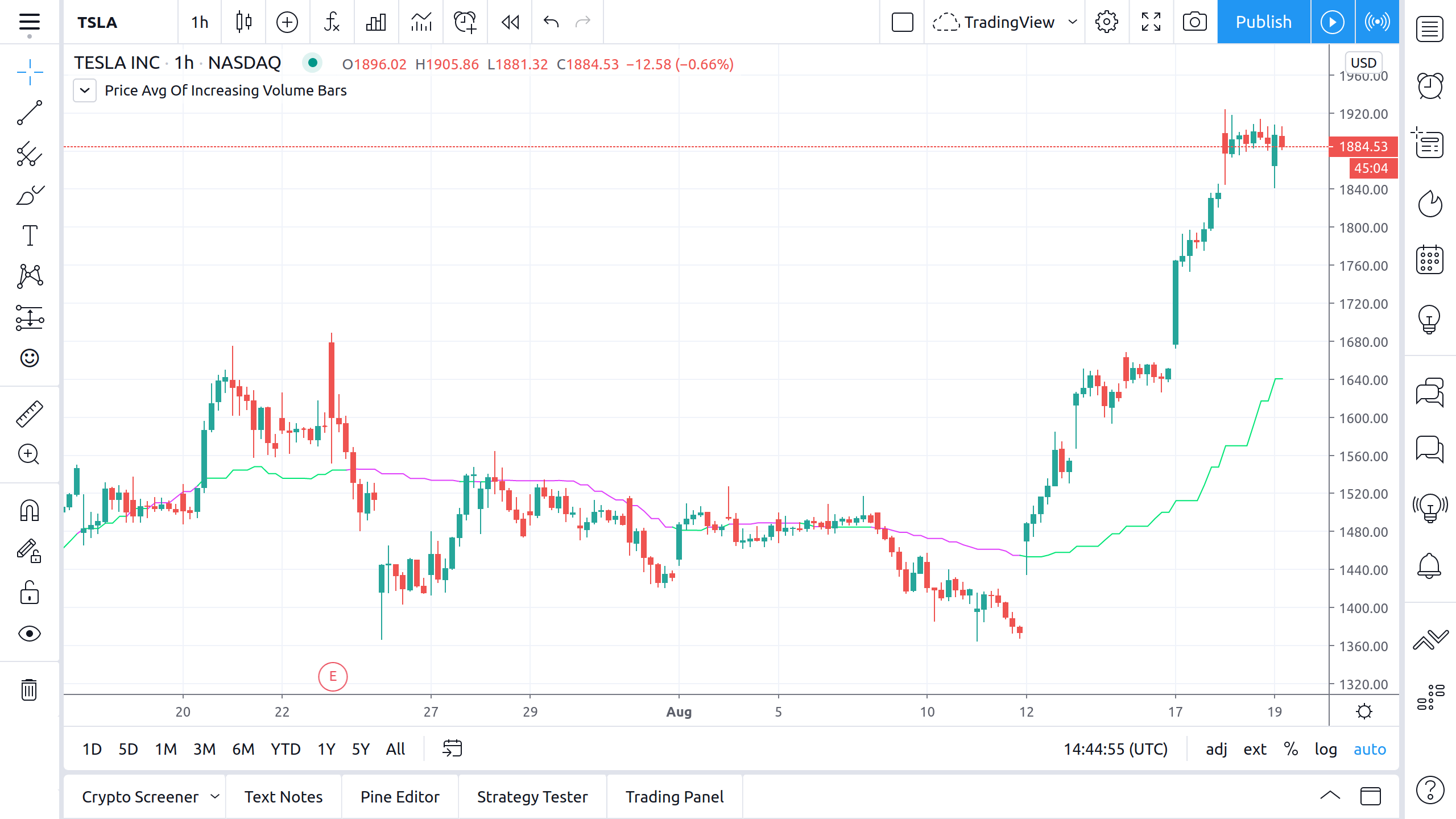Pine用户手册的阵列章节将帮助您入门。另外，请参阅PineCoders在这些脚本中发挥作用的阵列，他们在测试阵列方面为我们提供了最大帮助，我们由衷地感谢：RicardoSantosDuyck

Graph style – 4th Dimension RSI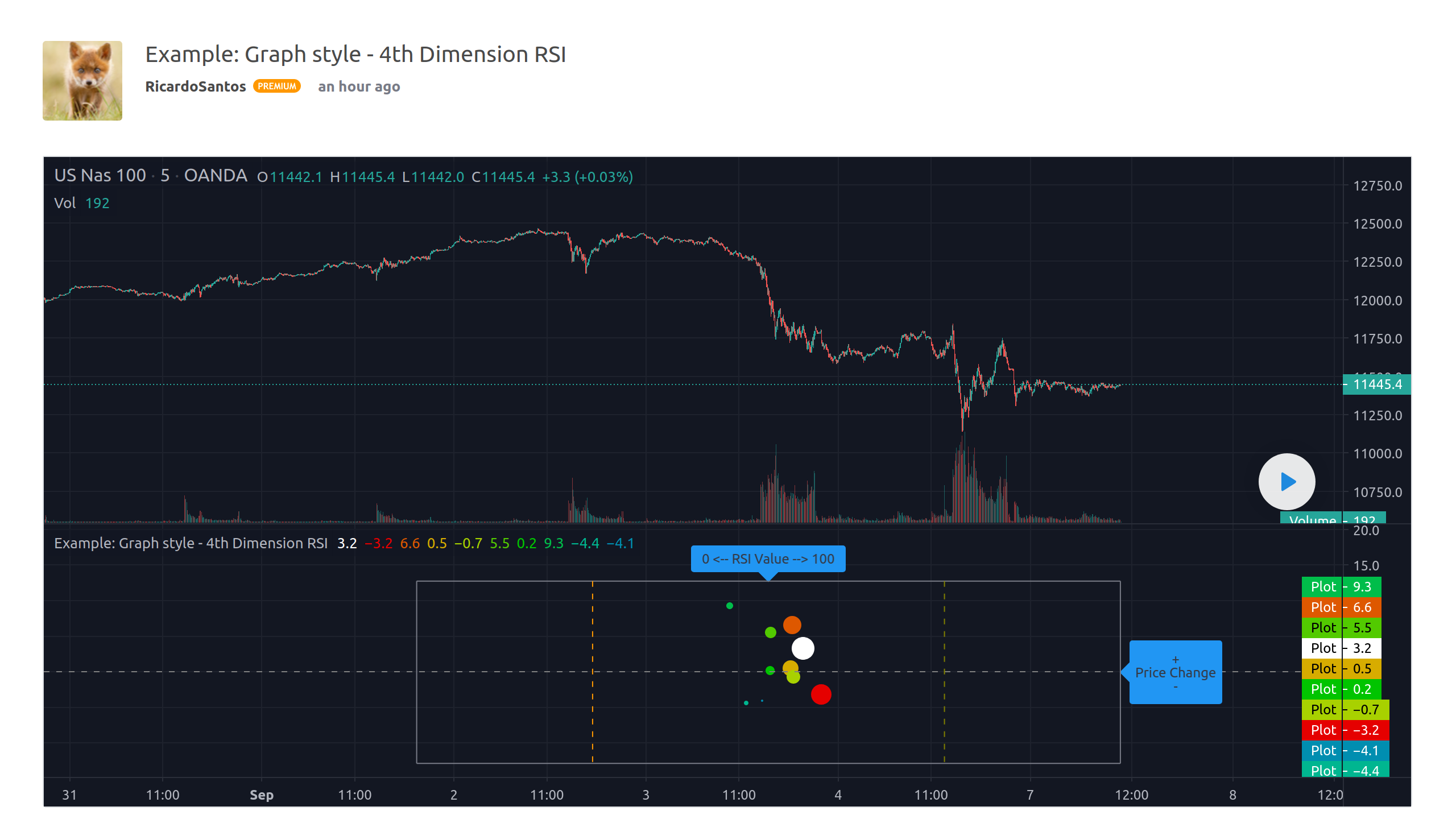Function – Multi Dimension IndexerFunction – K-Means Clustering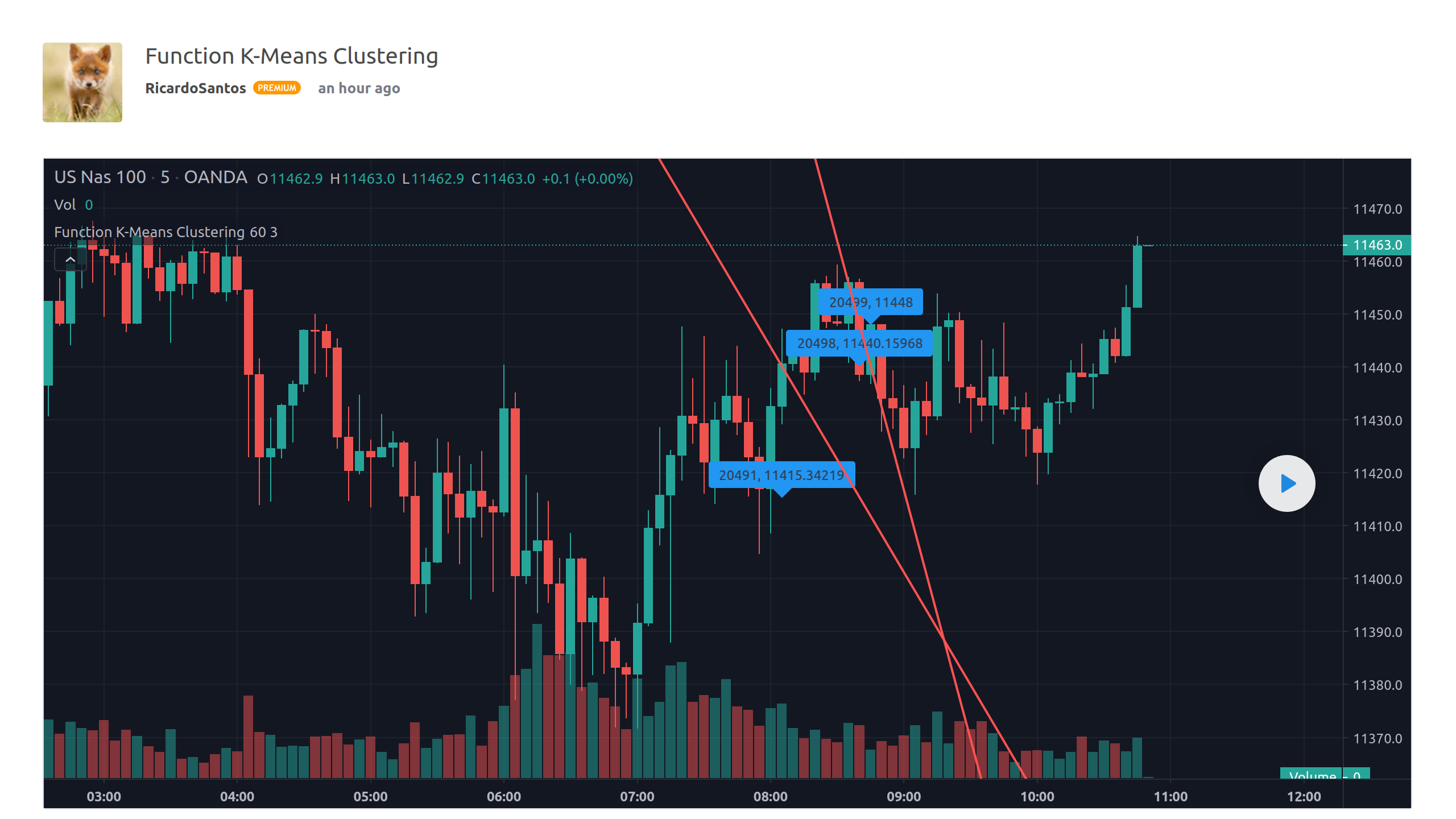Function – Linear RegressionFunction – Polynomial Regression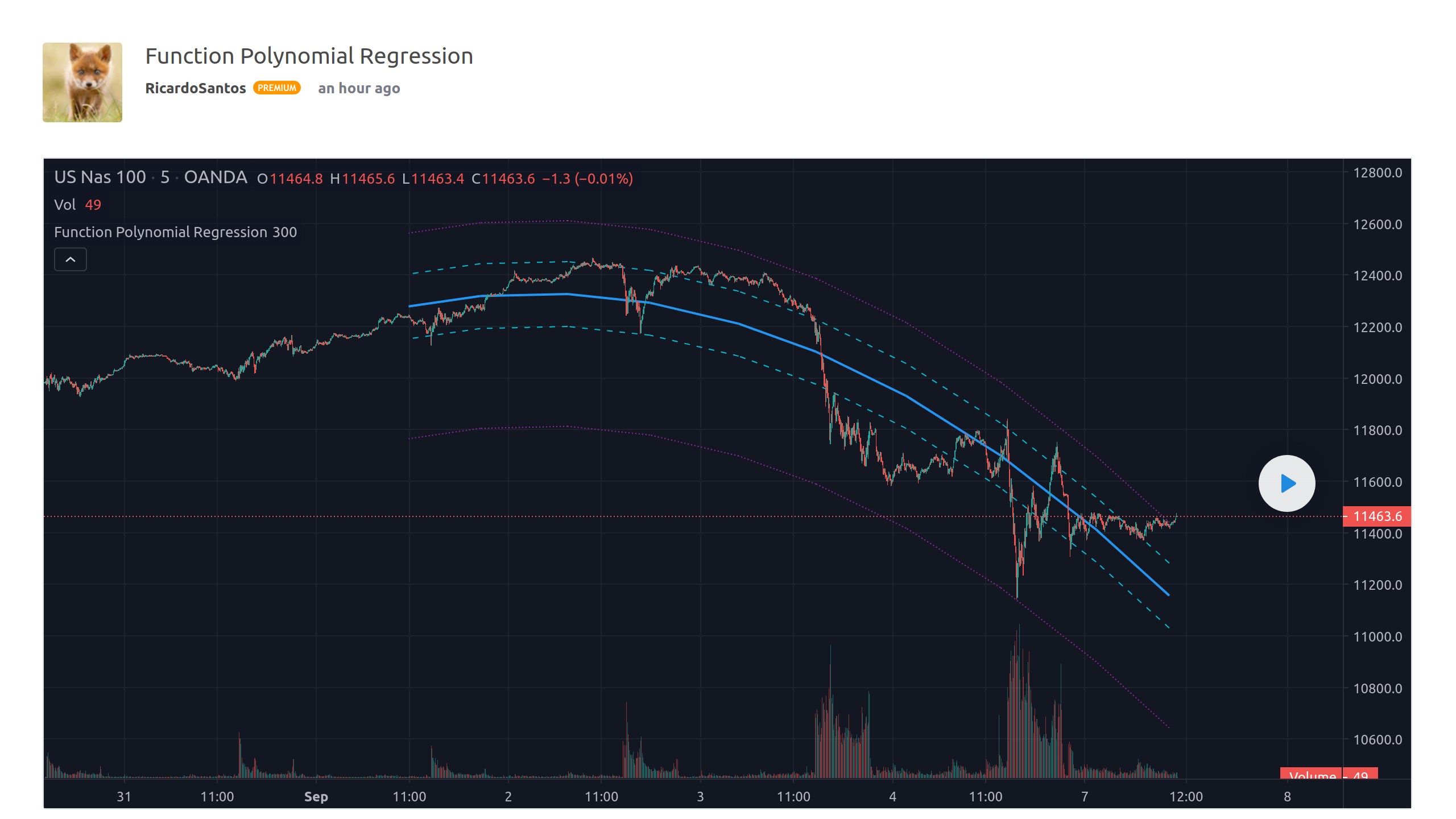Trendlines – JDAverage Pivot Range – JDMatrix functions – JD